Ohio EOCE Integrated Math I: Rational Expressions Chapter Exam

Exam Instructions:

Choose your answers to the questions and click 'Next' to see the next set of questions. You can skip questions if you would like and come back to them later with the yellow "Go To First Skipped Question" button. When you have completed the practice exam, a green submit button will appear. Click it to see your results. Good luck!

Page 1

Question 1 1. Solve for x.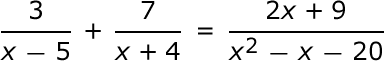Question 2 2. Add and simplify.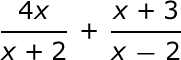Question 5 5. Solve.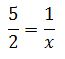Page 2

Question 7 7. Solve.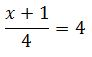Question 8 8. Subtract and simplify.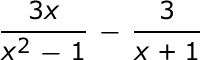Question 9 9. Multiply the following expressions: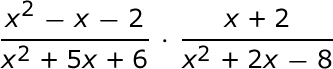Question 10 10. Solve.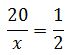Page 3

Question 11 11. What is the common denominator?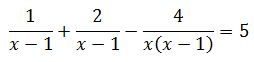Question 12 12. Multiply the following rational expressions.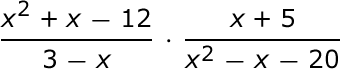Page 4

Question 16 16. Solve for x.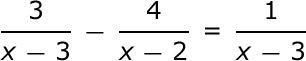Question 17 17. Divide the following expressions: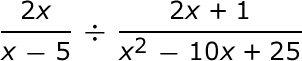Question 18 18. Solve for x.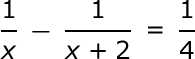Page 5

Question 21 21. Solve for x.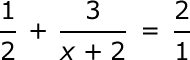Question 22 22. Solve the following rational equation.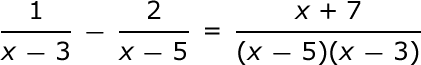Question 23 23. Solve the rational equation.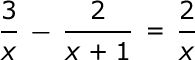Question 24 24. Divide the following rational expressions.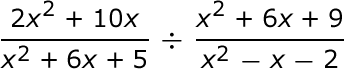Page 6

Question 26 26. Multiply the following rational expressions.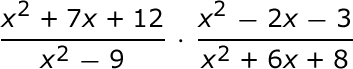Question 27 27. Subtract and simplify.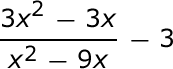Question 28 28. Solve for x.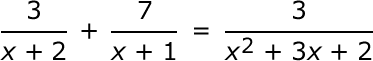Question 29 29. Add and simplify.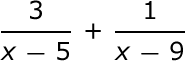Question 30 30. Add the following rational expressions.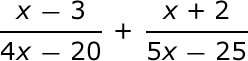Ohio EOCE Integrated Math I: Rational Expressions Chapter Exam Instructions

Choose your answers to the questions and click 'Next' to see the next set of questions. You can skip questions if you would like and come back to them later with the yellow "Go To First Skipped Question" button. When you have completed the practice exam, a green submit button will appear. Click it to see your results. Good luck!

Support